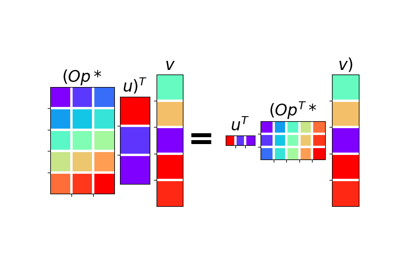pylops.utils.dottest¶

pylops.utils.dottest(Op, nr, nc, tol=1e-06, complexflag=0, raiseerror=True, verb=False)[source]

Dot test.

Generate random vectors $$\mathbf{u}$$ and $$\mathbf{v}$$ and perform dot-test to verify the validity of forward and adjoint operators. This test can help to detect errors in the operator implementation.

Parameters: Op : pylops.LinearOperator Linear operator to test. nr : int Number of rows of operator (i.e., elements in data) nc : int Number of columns of operator (i.e., elements in model) tol : float, optional Dottest tolerance complexflag : bool, optional generate random vectors with real (0) or complex numbers (1: only model, 2: only data, 3:both) raiseerror : bool, optional Raise error or simply return False when dottest fails verb : bool, optional Verbosity ValueError If dot-test is not verified within chosen tolerance.

Notes

A dot-test is mathematical tool used in the development of numerical linear operators.

More specifically, a correct implementation of forward and adjoint for a linear operator should verify the the following equality within a numerical tolerance:

$(\mathbf{Op}*\mathbf{u})^H*\mathbf{v} = \mathbf{u}^H*(\mathbf{Op}^H*\mathbf{v})$

Examples using pylops.utils.dottest¶02. The Dot-Test